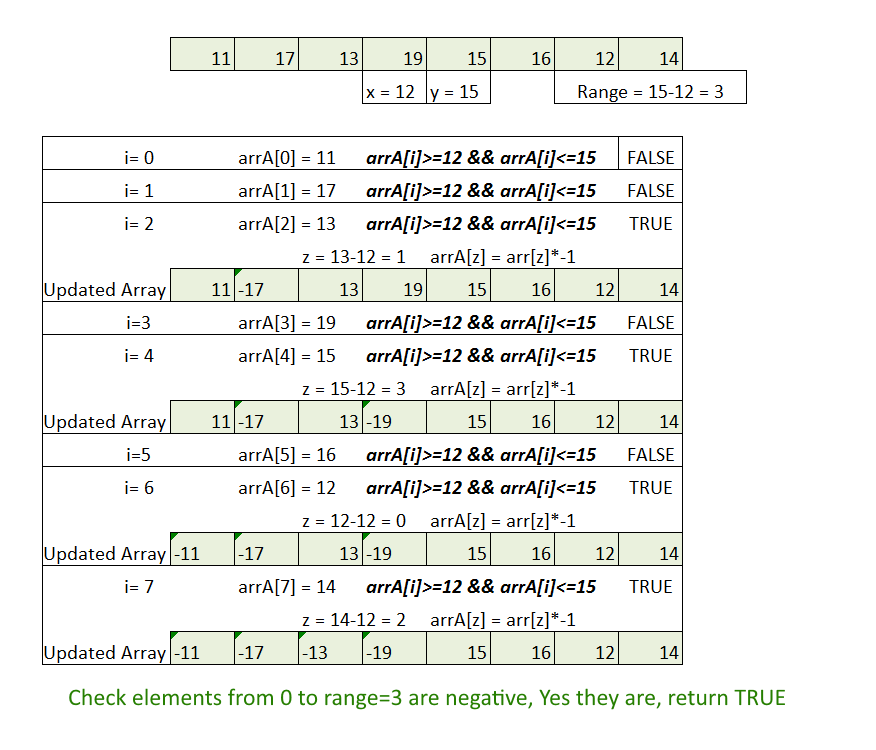Medium

# Check if Array Contains All Elements Of Some Given Range

Objective: Given an array of unsorted numbers, check if it contains all elements of some given range.

Examples:

```int[] arrA = { 11, 17, 13, 19, 15, 16, 12, 14 };
Range : 12-15
Output: True
```

Approach:

Naive Approach: Sorting . Time Complexity - O(nlogn).

Better Approach: Time Complexity - O(n).

• Find the range = y-x;
• Do the linear scan of array.
• Check if element falls within the range of x and y, (arrA[i]>=x && arrA[i]<=y)
• If Yes then calculate z = arrA[i]-x;
• Make the arrA[z] element as negative.
• Once the linear scan is done, just check all the elements in arrA[] from 0 to range are negative, if yes them array contains all the numbers of the given range, return true, else false.
• See the Picture below for better explanation

[caption id="attachment_629" align="aligncenter" width="665"]Check if Array Contains All Elements Of Some Given Range[/caption]

Code:

Output:

```True
```